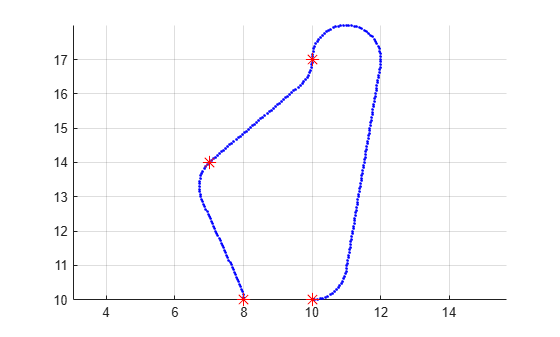Documentation

# navPath

Planned path

## Description

The `navPath` object stores paths that are typically created by geometric path planners. Path points are stored as states in an associated state space.

## Creation

### Syntax

``path = navPath``
``path = navPath(space)``
``path = navPath(space,states)``

### Description

````path = navPath` creates a path object, `path`, using the SE2 state space with default settings. ```

example

````path = navPath(space)` creates a path object with state space specified by `space`. The `space` input also sets the value of the StateSpace property.```
````path = navPath(space,states)` allows you to initialize the path with state samples given by `states`. Specify `states` as a matrix of state samples. States that are outside of the `StateBounds` of the state `space` object are reduced to the bounds. The `states` input also sets the value of the States property.```

## Properties

expand all

State space for the path, specified as a state space object. Each state in the path is a sample from the specified state space. You can use objects such as `stateSpaceSE2`, `stateSpaceDubins`, or `stateSpaceReedsShepp` as a state space object. You can also customize a state space object using the `nav.StateSpace` object.

Data Types: `object`

States of the path, specified as a real-valued M-by-N matrix. M is the number of states in the path, and N is the dimension of each state. You can only set this property during object creation or using the `append` function.

Data Types: `double`

Number of state samples in the path, specified as a nonnegative integer. The number is the same as the number of rows of the state matrix specified in the `States` property.

Data Types: `double`

## Object Functions

 `append` Add states to end of path `copy` Create copy of path object `interpolate` Interpolate points along path `pathLength` Length of path

## Examples

collapse all

Create a navPath object based on multiple waypoints in a Dubins space.

`dubinsSpace = stateSpaceDubins([0 25; 0 25; -pi pi])`
```dubinsSpace = stateSpaceDubins with properties: SE2 Properties Name: 'SE2 Dubins' StateBounds: [3x2 double] NumStateVariables: 3 Dubins Vehicle Properties MinTurningRadius: 1 ```
`pathobj = navPath(dubinsSpace)`
```pathobj = navPath with properties: StateSpace: [1x1 stateSpaceDubins] States: [0x3 double] NumStates: 0 ```
```waypoints = [... 8 10 pi/2; 10 12 pi/4; 12 17 pi/2; 11 10 -pi]; append(pathobj, waypoints);```

Interpolate that path so that it contains exactly 250 points.

`interpolate(pathobj, 250)`

Visualize the interpolated path and the original waypoints.

```figure; grid on; axis equal; hold on; plot(pathobj.States(:,1), pathobj.States(:,2), ".b"); plot(waypoints(:,1), waypoints(:,2), "*r", "MarkerSize", 10)```Calculate length of path.

```len = pathLength(pathobj); disp("Path length = " + num2str(len))```
```Path length = 19.37 ```